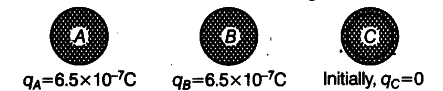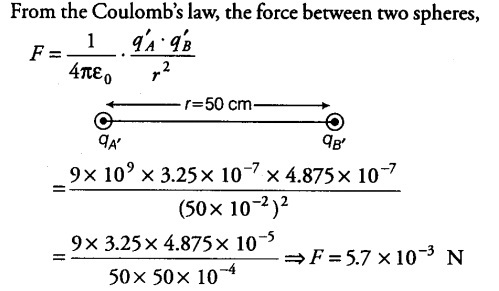# Suppose the spheres A and Bin Q. 2 have identical sizes

Suppose the spheres A and Bin Q. 2 have identical sizes. A third sphere of the same size - but uncharged is brought in contact with the first, then brought in contact with the second and finally removed from both. What is the new force of repulsion

Now,the sphere C becomes in contact with A , the charges will be divided equally on both spheres as they have same mass and size.Now, on charge A isq’a = qa+qc / 2 = 6.5 x {{10}^{-7}} + 0 /2 = 3.25 x {{10}^{-7}} C
Now,the charge on C
q’c = 3.25 x {{10}^{-7}} C
Now,sphere C contacts with B, the charges are shared again.
Now, charge on B,
q’b = qb+q’c/2 = 6.5 x {{10}^{-7}} + 3.25 x {{10}^{-7}} /2 = 4.875 x {{10}^{-7}} C
Finally, the charge on C = 4.875 x {{10}^{-7}} C
Finally, the charge on A = 3.25 x {{10}^{-7}} C
The charge on B = 4.875 x {{10}^{-7}} CThe force will be repulsive in nature because both spheres have like charges.